# Average per Week by Formula in Excel

We usually apply AVERAGE function or relevant functions to return average directly in Excel worksheet. But in some situations, only applying average relevant functions cannot figure out our problem. Sometimes we can create a formula with functions and mathematical operation inside to calculate average depends on different situations.

In this article, through explaining the example below, we will introduce you to apply SUMPRODUCT and COUNTIF functions together to calculate average. You can get the basic knowledge and usage of these two functions in this article.

## EXAMPLE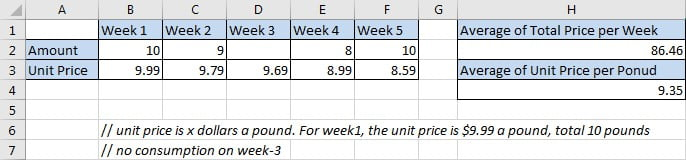The unit for “Amount” is pound. The unit for “Unit Price” is one dollar a pound. From the table we can know that unit prices are fluctuant every week. In different weeks, we bought different amounts of item per our demands. For example, on week-1, we bought 10 pounds with a unit price \$9.99, but on week-3, we didn’t pay for this item. Now, we want to know the average of our consumption per week, and the average of unit price we cost for five weeks.

In this article, to approach our goal, we will apply SUMPRODUCT function and COUNTIF function, and use an expression =SUMPRODUCT()/COUNTIF() to get the average of total price per week; and apply SUMPRODUCT function and SUM function, and expression =SUMPRODUCT()/SUM() to get the average of unit price for one pound during five weeks.

## ANALYSIS

To calculate “average of total price per week”:

a. In mathematics, the general formula to figure out this problem is “Average=(Total price of week1 + Total price of week2 + … + total price of week N)/N weeks”.

b. To calculate the total price of each week, we can create a simple formula that multiplies amount by unit price, for example, for week1, total price is B2*B3.

c The total price of all five weeks is equal to (B2*B3+C2*C3+…+F2*F3). Actually, this operation can be seen as two arrays multiplication that “values from range B2:F2 multiply the values from range B3:F3” correspondingly, then sum all products properly. Till now, we are sure we can apply Excel Math function “SUMPRODUCT” here to sum total price of five weeks simply.

d. For number of weeks, as there is no consumption on week-3, so week-3 should be excluded when counting number of weeks. As D2 is blank, so we can apply COUNT function directly to count weeks as COUNT function can ignore blank cell automatically. To make sense, we apply COUNTIF function instead of COUNT We want to count weeks with consumption, so amount should be greater than 0. To count with one condition or criterion, we choose COUNTIF function here.

e. Then we can create a formula =SUMPRODUCT(B2:F2*B3:F3)/COUNTIF(B2:F2,”>0”) to return average.

To calculate “average of unit price per pound”:

a. In mathematics, the general formula to figure out this problem is “Average=(Total price of week1 + Total price of week2 + … + total price of week N)/Total amounts”. We can repeat above first three steps to get sum of total prices.

b. For total amounts, just apply SUM function which is one of the most frequently used math functions in Excel.

c. Then we can create a formula =SUMPRODUCT(B2:F2*B3:F3)/SUM(B2:F2) to return average.

## FORMULA

Input formula =SUMPRODUCT(B2:F2*B3:F3)/COUNTIF(B2:F2,”>0″) into H2 and press Enter, verify that 86.46 is returned and shown in H2 properly. (10*9.99+9*9.79+8*8.99+10*8.59)/4=86.46. The return value is correct.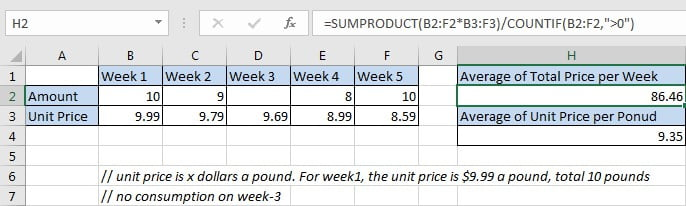Notice: actually, the returned value may be a number with more than two decimal places (based on your settings), you can click “Decrease Decimal” in “Number” section under Home tab to shorten decimal places and keep only two.

Input formula =SUMPRODUCT(B2:F2*B3:F3)/SUM(B2:F2) into H4 and press Enter, verify that 9.35 is returned and shown in H4 properly. (10*9.99+9*9.79+8*8.99+10*8.59)/ (10+9+8+10)=9.35. The return value is correct.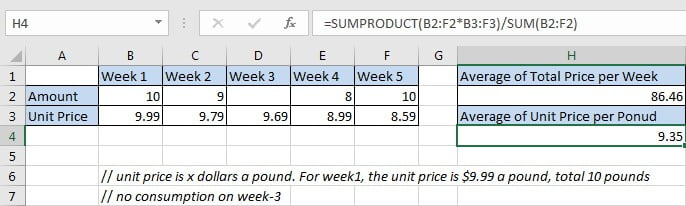## FUNCTION INTRODUCTION

a. SUMPRODUCT function returns the sum of the products of multiplication of multiple corresponding arrays or ranges.

Syntax:

`=SUMPRODUCT(array1, [array2], [array3],…)`

Example.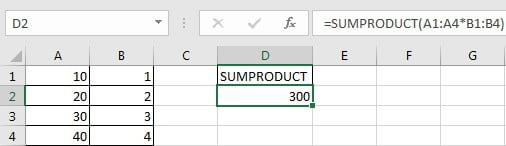b. COUNTIF returns the number of cells which contain a number and the number meets one condition or criterion. The condition or criterion is determined by our input in “criteria” argument.

Syntax:

` =COUNTIF(range, criteria)`

Example.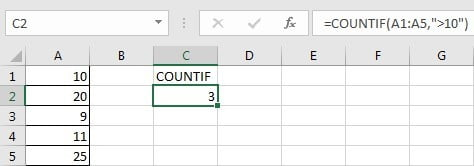c. SUM function returns sum of a set of numbers.

Syntax:

`=SUM(number1,[number2], …)`

Example.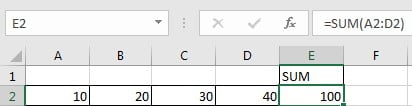## EXPLANATION

`=SUMPRODUCT(B2:F2*B3:F3)/COUNTIF(B2:F2,">0")`

// for SUMPRODUCT, array1 is B2:F2*B3:F3

// for COUNTIF, range is B2:F2, criteria “>0”

a. SUMPRODUCT(B2:F2*B3:F3) returns the sum of total prices of all five weeks. Expand values in B2:F2 and B3:F3, we get below expression:

`=SUMPRODUCT({10,9,0,8,10}*{9.99,9.79,9.69,8.99,8.59}) //`

returns 345.83

b. COUNTIF(B2:F2,”>0″) returns number of weeks with consumption. Expand values from range B2:F2, we get below expression:

`=COUNTIF({10,9,0,8,10},">0")//`

returns “4”

c. Above all, =SUMPRODUCT(B2:F2*B3:F3)/COUNTIF(B2:F2,”>0″) returns 345.83/4=86.46.

`=SUMPRODUCT(B2:F2*B3:F3)/SUM(B2:F2)`

// for SUMPRODUCT, array1 is B2:F2*B3:F3

// for SUM, numbers are saved in B2:F2

a. SUM(B2:F2) returns the sum of total amounts of all five weeks. Expand values in B2:F2, we get below expression:

`=SUM({10,9,0,8,10}) //`

returns 37

b. As SUMPRODUCT function returns 345.83, so =SUMPRODUCT(B2:F2*B3:F3)/COUNTIF(B2:F2,”>0″) returns 345.83/37=9.35.

### Related Functions

• Excel SUM function
The Excel SUM function will adds all numbers in a range of cells and returns the sum of these values. You can add individual values, cell references or ranges in excel.The syntax of the SUM function is as below:= SUM(number1,[number2],…)…
• Excel SUMPRODUCT function
The Excel SUMPRODUCT function multiplies corresponding components in the given one or more arrays or ranges, and returns the sum of those products.The syntax of the SUMPRODUCT function is as below:= SUMPRODUCT (array1,[array2],…)…
• Excel COUNTIF function
The Excel COUNTIF function will count the number of cells in a range that meet a given criteria. This function can be used to count the different kinds of cells with number, date, text values, blank, non-blanks, or containing specific characters.etc.= COUNTIF (range, criteria)…
• Excel AVERAGE function
The Excel AVERAGE function returns the average of the numbers that you provided.The syntax of the AVERAGE function is as below:=AVERAGE (number1,[number2],…)….